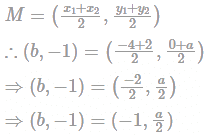Point Q(b,−1) is the midpoint of segment EF....
Point Q(b,−1) is the midpoint of segment EF. Co-ordinates of point E are (−4, a) and point F are (2,0). What is the value of a and b?
Point Q(b,−1) is the midpoint of segment EF. Co-ordinates of point E ...
Given,
Point Q(b,−1) is the midpoint of segment with endpoints E(−4, a) and F(2, 0).
As we know,
The midpoint of a segment with endpoints (x1, y1) and (x2, y2) is given by,On comparing, we get
b = −1, a = −2
Hence, the correct answer is a = -2, b = -1.
 1 Crore+ students have signed up on EduRev. Have you?
1 Crore+ students have signed up on EduRev. Have you?

### Learn this topic in detailIPMAT Mock Test - 9 (New Pattern) 100 Ques | 90 MinsMore from Related Course Quantitative Aptitude (Quant)View courses related to this question

### Quick links for CAT exam850+
Video Lectures2500+
Revision Notes600+
Online Tests10,000+
Doubts Solved
Point Q(b,−1) is the midpoint of segment EF. Co-ordinates of point E are (−4, a) and point F are (2,0). What is the value of a and b?Correct answer is '-2, -1'. Can you explain this answer?
Question Description
Point Q(b,−1) is the midpoint of segment EF. Co-ordinates of point E are (−4, a) and point F are (2,0). What is the value of a and b?Correct answer is '-2, -1'. Can you explain this answer? for CAT 2023 is part of CAT preparation. The Question and answers have been prepared according to the CAT exam syllabus. Information about Point Q(b,−1) is the midpoint of segment EF. Co-ordinates of point E are (−4, a) and point F are (2,0). What is the value of a and b?Correct answer is '-2, -1'. Can you explain this answer? covers all topics & solutions for CAT 2023 Exam. Find important definitions, questions, meanings, examples, exercises and tests below for Point Q(b,−1) is the midpoint of segment EF. Co-ordinates of point E are (−4, a) and point F are (2,0). What is the value of a and b?Correct answer is '-2, -1'. Can you explain this answer?.
Solutions for Point Q(b,−1) is the midpoint of segment EF. Co-ordinates of point E are (−4, a) and point F are (2,0). What is the value of a and b?Correct answer is '-2, -1'. Can you explain this answer? in English & in Hindi are available as part of our courses for CAT. Download more important topics, notes, lectures and mock test series for CAT Exam by signing up for free.
Here you can find the meaning of Point Q(b,−1) is the midpoint of segment EF. Co-ordinates of point E are (−4, a) and point F are (2,0). What is the value of a and b?Correct answer is '-2, -1'. Can you explain this answer? defined & explained in the simplest way possible. Besides giving the explanation of Point Q(b,−1) is the midpoint of segment EF. Co-ordinates of point E are (−4, a) and point F are (2,0). What is the value of a and b?Correct answer is '-2, -1'. Can you explain this answer?, a detailed solution for Point Q(b,−1) is the midpoint of segment EF. Co-ordinates of point E are (−4, a) and point F are (2,0). What is the value of a and b?Correct answer is '-2, -1'. Can you explain this answer? has been provided alongside types of Point Q(b,−1) is the midpoint of segment EF. Co-ordinates of point E are (−4, a) and point F are (2,0). What is the value of a and b?Correct answer is '-2, -1'. Can you explain this answer? theory, EduRev gives you an ample number of questions to practice Point Q(b,−1) is the midpoint of segment EF. Co-ordinates of point E are (−4, a) and point F are (2,0). What is the value of a and b?Correct answer is '-2, -1'. Can you explain this answer? tests, examples and also practice CAT tests.
Do you know? How Toppers prepare for CAT Exam
With help of the best CAT teachers & toppers, We have prepared a guide for student who are preparing for CAT : 15 Steps to clear CAT Exam(Scan QR code)